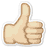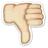Meaning Frequency polygon
What does Frequency polygon mean? Here you find 6 meanings of the word Frequency polygon. You can also add a definition of Frequency polygon yourself

# 1

11# Frequency polygon

A graph of a frequency distribution obtained by drawing straight lines joining successive points representing the class frequencies.
 Source: cfainstitute.org

# 2

11# Frequency polygon

a line graph which joins the midpoints of the top of the bars on a frequency histogram. • a frequency polygon gives a picture of the shape of the data distribution.
 Source: amathsdictionaryforkids.com

# 3

11# Frequency polygon

the name given to the graph that depicts a statistical distribution that is made up of lines connecting the peaks of adjacent intervals.
 Source: psychologydictionary.org

# 4

00# Frequency polygon

A frequency polygon is a line graph made by connecting the top center scores of the columns of a frequency histogram. A frequency histogram is a bar graph that uses vertical columns to visually show f [..]
 Source: alleydog.com

# 5

11# Frequency polygon

A graph formed by joining the midpoints of histogram column tops. (See also: histogram ).
 Source: statcan.gc.ca

# 6

01# Frequency polygon

A graph of a frequency distribution with values of the variable on the x-axis and the number of observations on the y-axis; data points are plotted at the midpoints of the intervals and are connected [..]
 Source: cs.columbia.edu

 Add meaning of Frequency polygon Word count:
 Name: E-mail: (* optional)

 << Free cash flow to equity Foundations >>

Dictionary.university is a dictionary written by people like you and me.